• Save

# Pattern Completion Questions Non Verbal Reasoning for Competitive Exams

2 weeks ago 3.1K Views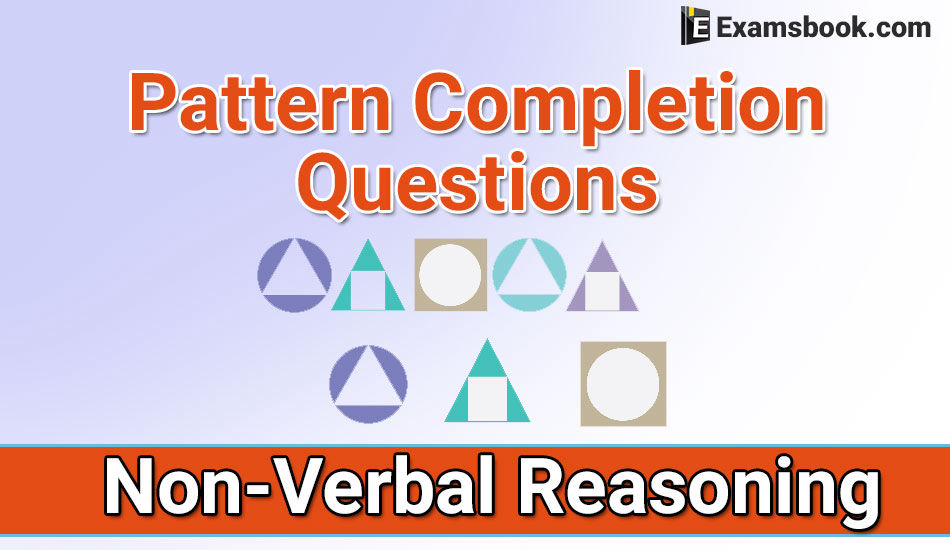There is the important role of pattern completion questions non-verbal reasoning in competitive exams. So, every student wants to find that place where they can practice better for their exams because they want to prepare without wasting their time.

So, here students can get pattern completion questions non-verbal reasoning and improve their performance with practice these pattern completion questions without wasting time. This topic is very useful for those who doing preparation for competitive exams.

## Pattern Completion Questions and Answers Non-Verbal Reasoning:

Q.1. In the following question, which answer figure will complete the question figure?

Question figure:(A)                                  (B)                                  (C)                                 (D)

Ans .   C

Q.2. In the following question, which answer figure will complete the question figure?

Question figure: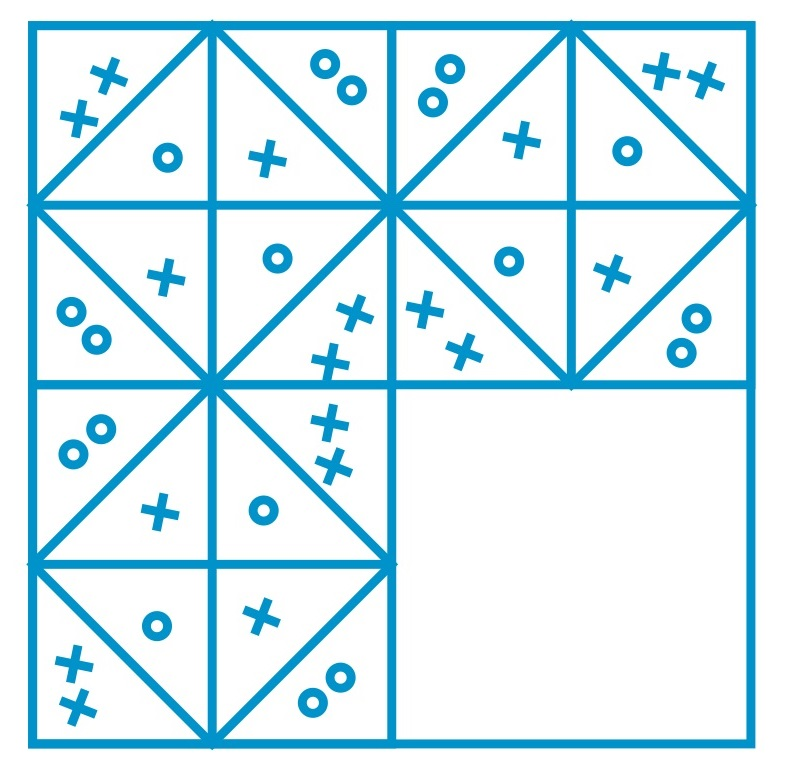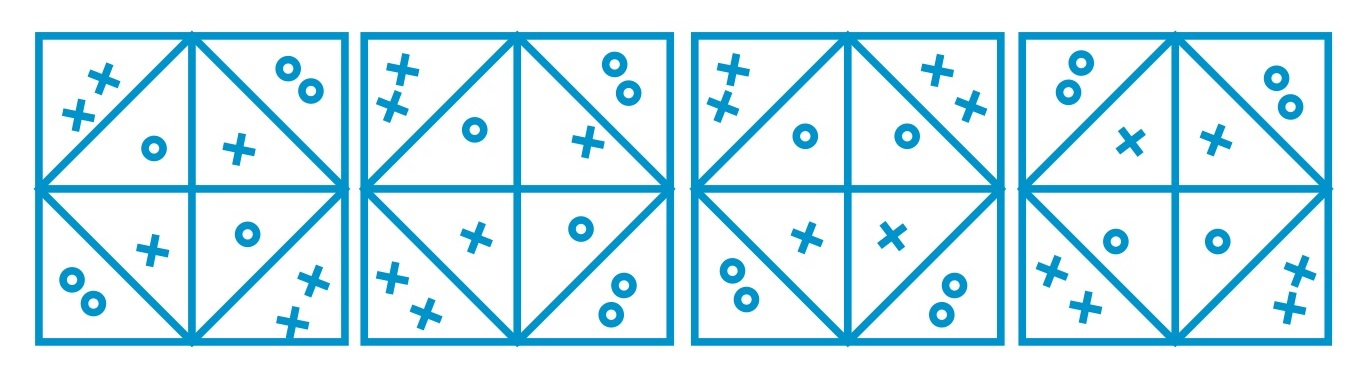(A)                              (B)                              (C)                              (D)

Ans .   A

Q.3.In the following question, which answer figure will complete the question figure?

Question figure: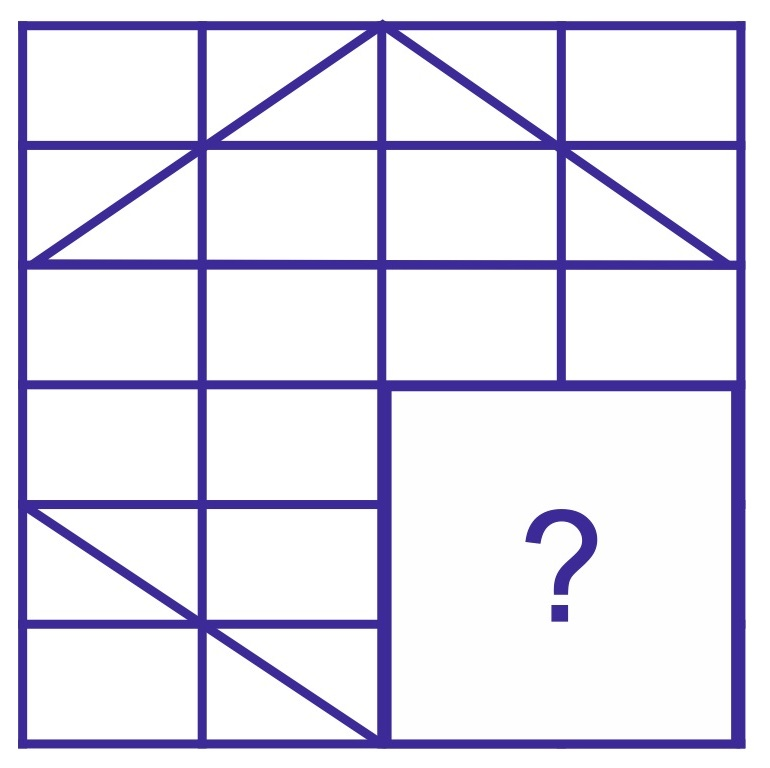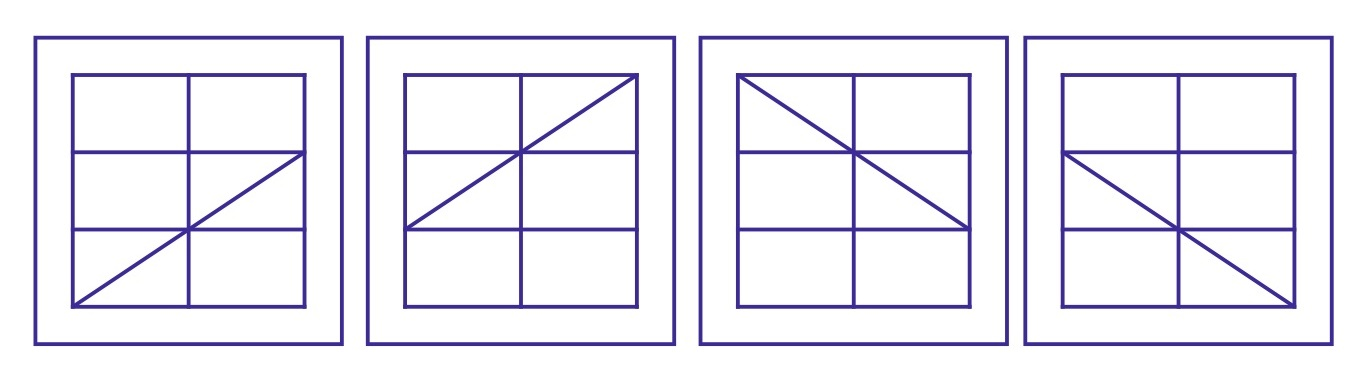(A)                                           (B)                                         (C)                                         (D)

Ans .   A

Q.4. In the following question, which answer figure will complete the question figure?

Question figure: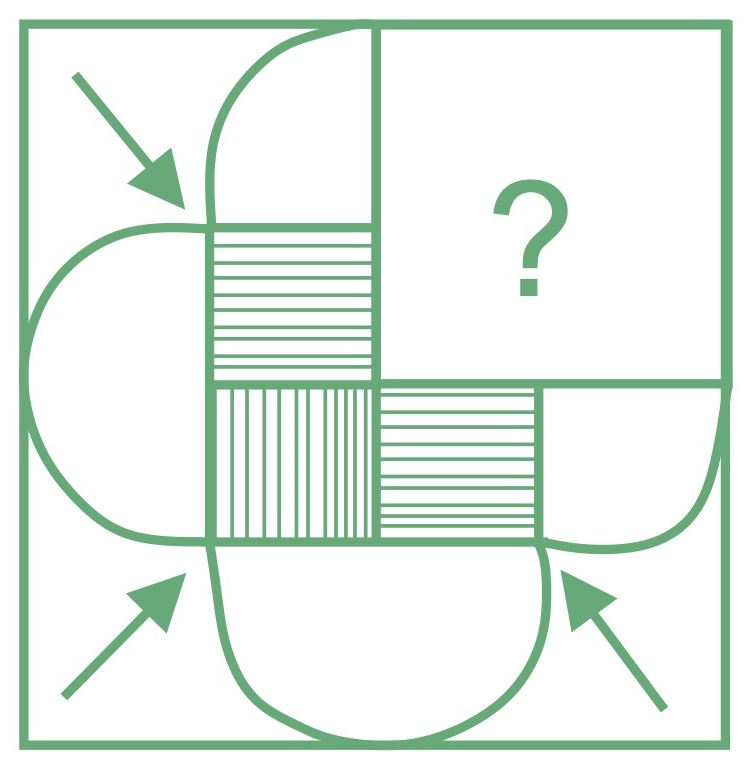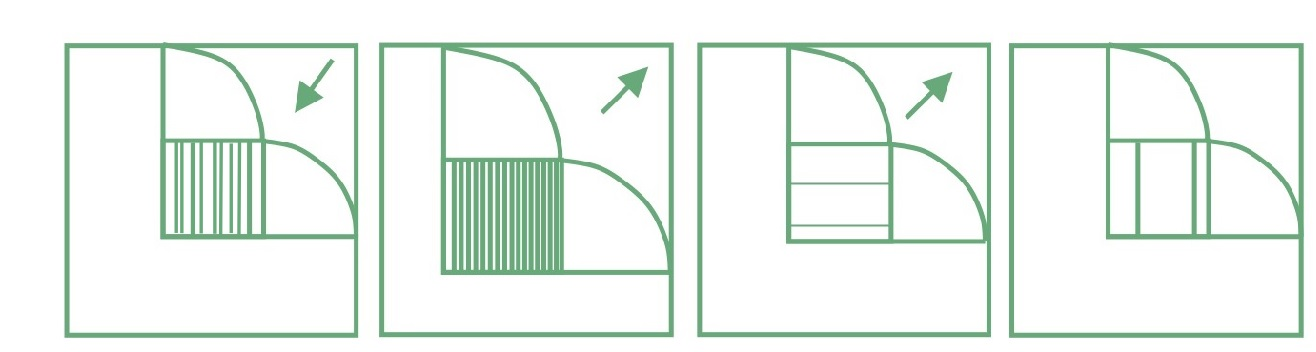(A)                                   (B)                                  (C)                                (D)

Ans .   A

Q.5. In the following question, which answer figure will complete the question figure?

Question figure: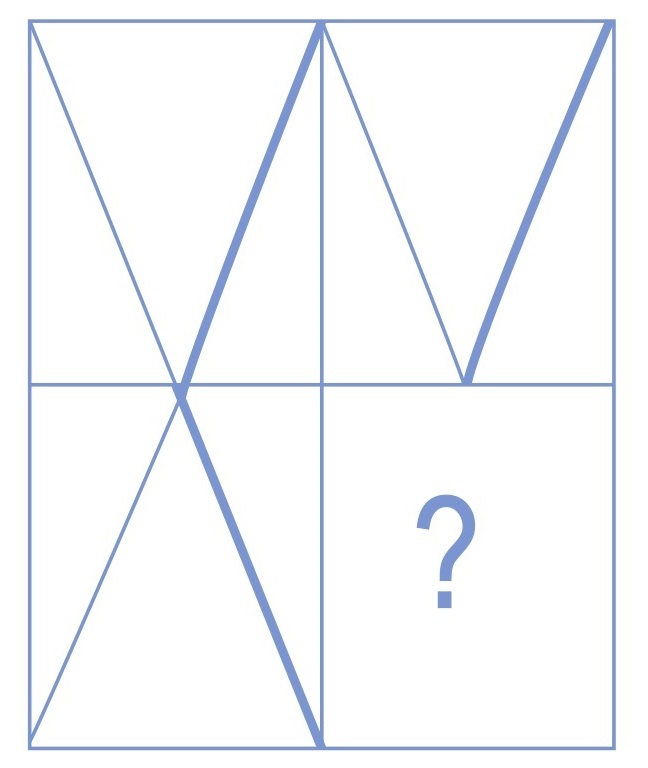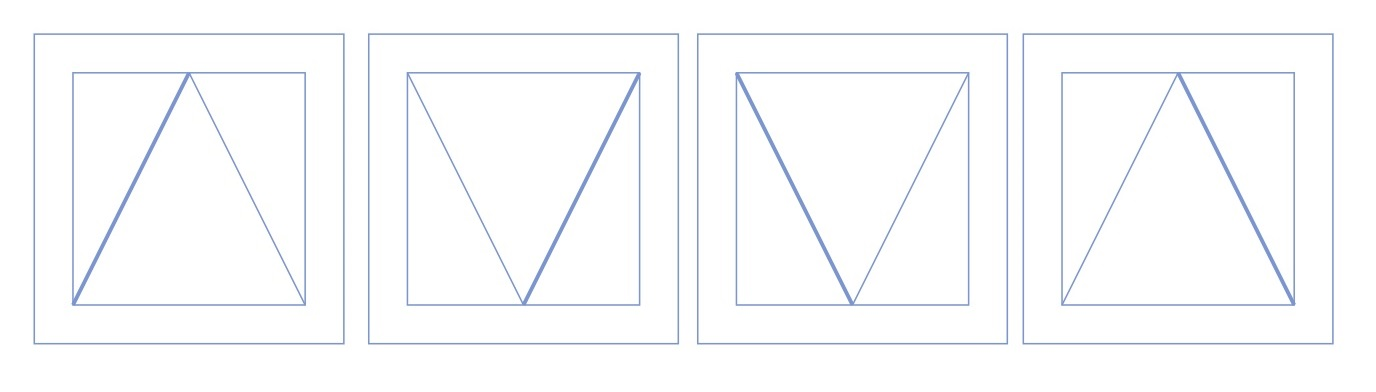(A)                              (B)                            (C)                           (D)

Ans .   D

Q.6. In the following question, which answer figure will complete the question figure?

Question figure: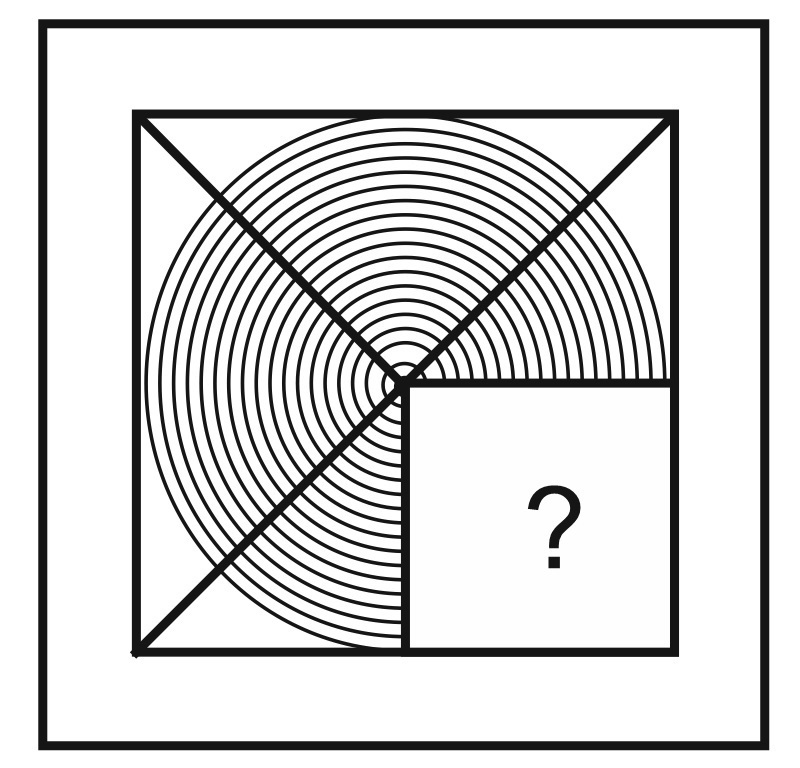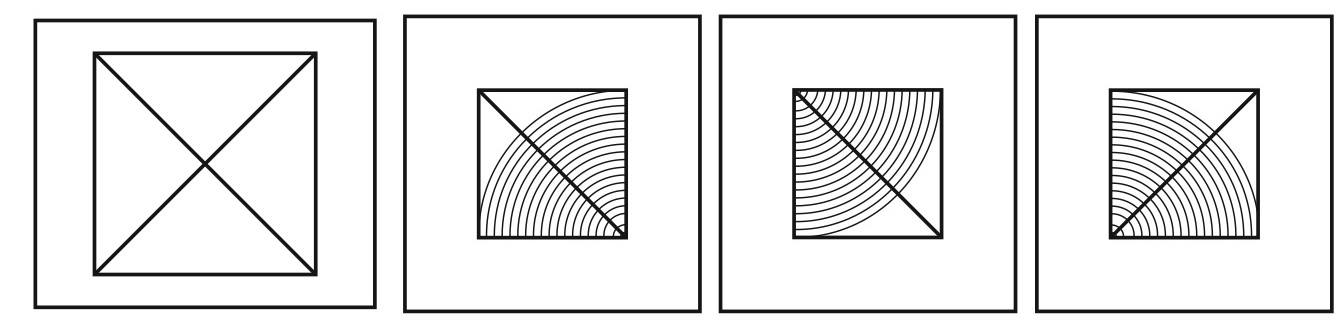(A)                                  (B)                              (C)                                (D)

Ans .   C

Comment or ask anything related to pattern completion questions non-verbal reasoning in the comment section. To more practice, pattern completion questions non-verbal reasoning questions and answers visit next page.